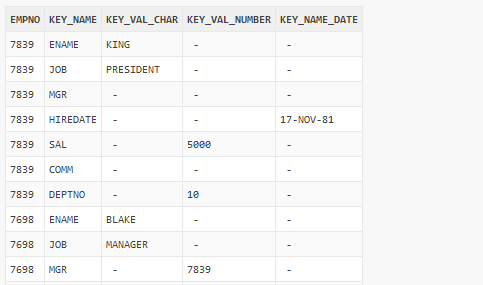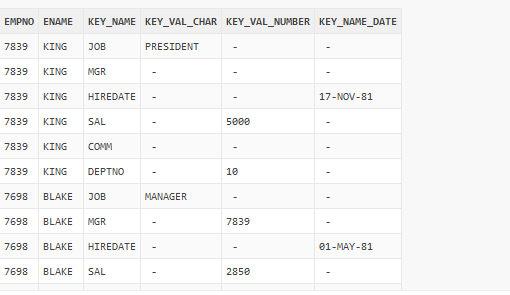# Polymorphic Table Functions Example – Transposing Columns To Rows

Hey, Oracle 18c is now available on the cloud and for engineered systems! For more than a week now. That also means you can play with it at LiveSQL. And of course you can try polymorphic table functions (PTF)! At least I’ve done that this weekend 😉 And here is my first working example.

I already mentioned in my first post on PTF, it would be nice to try to make PIVOT/UNPIVOT functions more dynamic. We’ll start with Unpivot or transposing columns to rows here. Let’s make a key-value store out of the `SCOTT.EMP` table. That means we’ll retain some identifying column(s), in our case this will be `EMPNO`. All other columns will be „packed“ using KEY_NAME-KEY_VALUE pairs. Maybe it will be a good idea not to use strings for all values, but to distinguish some data types. For our example, we will separate values of NUMBER, VARCHAR2 and DATE data types. So our target data structure will look something like this:

```EMPNO           NUMBER
KEY_NAME        VARCHAR2
KEY_VAL_CHAR    VARCHAR2
KEY_VAL_NUM     NUMBER
KEY_VAL_DATE    DATE
```

Let’s start coding. We’ll create a function TAB2KEYVAL which will accept the table and a list of ID-columns as parameter. The implementation of a polymorphic table function resides in a package. We must provide the function DESCRIBE which will be invoked during SQL cursor compilation and describes the shape of the returned result set. This function accepts the record type TABLE_T, a collection of columns COLUMNS_T and returns a recod type DESCRIBE_T. During the execution stage the rows are processed inside of the function FETCH_ROWS.

```CREATE OR REPLACE PACKAGE tab2keyval_pkg AS

FUNCTION describe(tab  IN OUT DBMS_TF.TABLE_T,
id_cols IN DBMS_TF.COLUMNS_T)
RETURN DBMS_TF.DESCRIBE_T;

PROCEDURE fetch_rows;

END tab2keyval_pkg;
/
```

That’s what we need for now and we can start the implementation of a package body. In the function DESCRIBE we are looping through all columns or the source table and mark them not to be passed through, unless this is the specified ID-column (lines 9 to 19). We also specify that all columns have to be processed during fetch phase (for_read = true). Again, unless it is an ID-column. Next we specify four new columns to hold the key name along with three possible key values (lines 22 to 29). That’s it. We just need to return a record DESCRIBE_T containing the information about new columns. Another point here: we are going to replicate the source rows, because we’ll get one row per transposed column (line 32).

Now we are ready to look at the processing itself – procedure FETCH_ROWS. Using procedure GET_ROW_SET (line 48), we get an input rowset with column values of the columns, which we had marked as FOR_READ. The returned datatype is a table or a record type COLUMN_DATA, which in turn has so called „variant fields“ – a tables for every supported datatype, e.g. tab_varchar2, tab_number and so on. Only one variant field corresponding to the actual datatype is active for a column.

Every row in a rowset has it’s own replication factor. We set it initially to 0 (lines 50 to 52). Then we loop through all rows and columns increasing the replication factor and filling the tables which hold the data for key_name and key_values with actual data. These tables – one for the key name (NAMECOL) and three for key values (VALCHARCOL, VALNUMCOL, VALDATECOL) – are defined at lines 43 to 46. The processing actually differs only slightly because of the different data types.

Next we specify the replication and pass the prepared collection for new columns back – the calls of PUT_COL at lines 78 to 82.

```CREATE OR REPLACE PACKAGE BODY tab2keyval_pkg AS
FUNCTION describe(tab  IN OUT DBMS_TF.TABLE_T,
id_cols IN DBMS_TF.COLUMNS_T)
RETURN DBMS_TF.DESCRIBE_T
AS
new_cols DBMS_TF.COLUMNS_NEW_T;
col_id   PLS_INTEGER := 1;
BEGIN
FOR I IN 1 .. tab.COLUMN.COUNT LOOP
tab.column(i).pass_through := FALSE;
-- Unless this is one of ID-columns
FOR J IN 1 .. id_cols.COUNT LOOP
IF ( tab.COLUMN(i).description.name = id_cols(j) ) THEN
tab.column(i).pass_through := TRUE;
END IF;
END LOOP;
END LOOP;

-- new columns: key_name, key_val_char, key_val_num, key_val_date
TYPE => dbms_tf.type_varchar2);
TYPE => dbms_tf.type_varchar2);
TYPE => dbms_tf.type_number);
TYPE => dbms_tf.type_date);

RETURN DBMS_TF.DESCRIBE_T(new_columns => new_cols
, row_replication=>true);
END;

PROCEDURE fetch_rows IS
inp_rs DBMS_TF.row_set_t;

env    dbms_tf.env_t := dbms_tf.get_env();

colcnt PLS_INTEGER;
rowcnt PLS_INTEGER;
repfac dbms_tf.tab_naturaln_t;
namecol dbms_tf.tab_varchar2_t;
valnumcol dbms_tf.tab_number_t;
valcharcol dbms_tf.tab_varchar2_t;
valdatecol dbms_tf.tab_date_t;
BEGIN
DBMS_TF.get_row_set(inp_rs, rowcnt, colcnt);

FOR i IN 1 .. rowcnt LOOP
repfac(i) := 0;
END LOOP;

FOR r IN 1 .. rowcnt LOOP
FOR c IN 1 .. colcnt LOOP
IF env.get_columns(c).type = dbms_tf.type_number then
repfac(r)                    := repfac(r) + 1;
namecol(nvl(namecol.last+1,1)) := replace(env.get_columns(c).name,'"');
valnumcol(NVL(valnumcol.last+1,1)) := inp_rs(c).tab_number(r);
valcharcol(NVL(valcharcol.last+1,1)) := NULL;
valdatecol(NVL(valdatecol.last+1,1)) := NULL;
ELSIF env.get_columns(c).type = dbms_tf.type_varchar2 then
repfac(r)                    := repfac(r) + 1;
namecol(nvl(namecol.last+1,1)) := replace(env.get_columns(c).name,'"');
valcharcol(NVL(valcharcol.last+1,1)) := inp_rs(c).tab_varchar2(r);
valnumcol(NVL(valnumcol.last+1,1)) := NULL;
valdatecol(NVL(valdatecol.last+1,1)) := NULL;
ELSIF env.get_columns(c).type = dbms_tf.type_date then
repfac(r)                    := repfac(r) + 1;
namecol(nvl(namecol.last+1,1)) := replace(env.get_columns(c).name,'"');
valdatecol(NVL(valdatecol.last+1,1)) := inp_rs(c).tab_date(r);
valcharcol(NVL(valcharcol.last+1,1)) := NULL;
valnumcol(NVL(valnumcol.last+1,1)) := NULL;
END IF;
END LOOP;
END LOOP;

dbms_tf.row_replication(replication_factor => repfac);
dbms_tf.put_col(1, namecol);
dbms_tf.put_col(2, valcharcol);
dbms_tf.put_col(3, valnumcol);
dbms_tf.put_col(4, valdatecol);

END;

END tab2keyval_pkg;
/

```

The package is there, so just define the function using it and it works!

```
CREATE OR REPLACE FUNCTION tab2keyval(tab TABLE,  id_cols COLUMNS)
RETURN TABLE PIPELINED ROW POLYMORPHIC USING tab2keyval_pkg;

SELECT  *
FROM    tab2keyval(scott.emp, COLUMNS(empno));

```What if we wanted to also use ENAME as identifying column and not to pack it in our key-value store? Just add it to COLUMNS operator. By the way, this COLUMNS SQL-operator is new in 18c and is only allowed in the parameter context of a polymorphic function. As we can see, the column ENAME is now a simple column in the output and not the part of key-value store any more (there is no rows with key_name = ‚ENAME‘ any more)

```
SELECT  *
FROM    tab2keyval(scott.emp, COLUMNS(empno, ename));

```That was quite simple! Now that we have produced this output, I’ll try to compose a PTF doing opposite task: transpose rows to columns, i.e. back to the original EMP table. In the next post. Maybe next weekend 😉